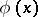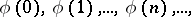# Infinite induction

(diff) ← Older revision | Latest revision (diff) | Newer revision → (diff)

Carnap's rule,-rule

A non-elementary derivation rule with an infinite number of premises. More exactly, let the variablein some logico-mathematical language be regarded as ranging over the natural numbers, and letbe a formula of this language. If each formula in the infinite listcan be derived, the rule of infinite induction permits to conclude that the formulais derivable as well.

The use of the rule of infinite induction in deriving formulas usually renders the problem of existence of a derivation undecidable. An axiomatic system containing an-rule is called a semi-formal theory (semi-formal axiomatic system). They play an important role in proof theory. In order to render the concept of a derivation in the theory effective, additional restrictions must be imposed to ensure that the premises can be effectively derived. It may be required, for example, that derivations of the premises are enumerated by some general recursive function (the so-called constructive rule of infinite induction). It is known that formal arithmetic (cf. Arithmetic, formal), completed by the constructive rule of infinite induction, is complete with respect to classical truth. The rule of infinite induction has also found use in constructing semantics of constructive mathematics by the method of stepwise semantic systems.

Still another term for the-rule is rule of complete induction.